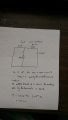# Test Question About Finding Time Constant with Dependent Source

#### bluetooth tamer

Joined Jan 16, 2015
23
Hi,

I just got back a test from lecture last week where one of the questions asks to find the time constant when the circuit has a dependent voltage source of 0.5k*ix and 2 resistors each 2k Ohm. My answer is 40ms but it got marked wrong.

Any help tackling this problem or showing me my error would be greatly appreciated.
Here's the picture redrawn for clarity.

Keep in mind that I make the assumption that this circuit is in DC steady state and this is not stated in the problem.

My logic from the picture in text goes like this.
The term ix = 0 because the cap is an open circuit. This makes the dependent source = 0, therefore the middle branch is a short and the resistance seen by the cap is 2k ohm. RC = 2k Ohm * 20 micro Farads.#### WBahn

Joined Mar 31, 2012
25,776
Your problem is that you can't treat dependent sources like you do independent ones. When you are finding the Thevenin equivalent circuit seen by the capacitor, you have to recognize that the dependent source matters.

#### bluetooth tamer

Joined Jan 16, 2015
23
Okay, I used Thevenin's equivalent and here's what I got:

If I plug in a voltage source of 1V in place of the cap then I can do a KVL around the right loop.
The equation I get is 1V = 2k*ix + 0.5k*ix where 1V/ix = 2.5k Ohms.
Multiply this resistance with capacitance and the answer is 50ms.

Is the correct way to approach such a problem?

#### WBahn

Joined Mar 31, 2012
25,776
Yep. Good job.

Notice how the dependent voltage source completely masks the left hand resistor.

•bluetooth tamer